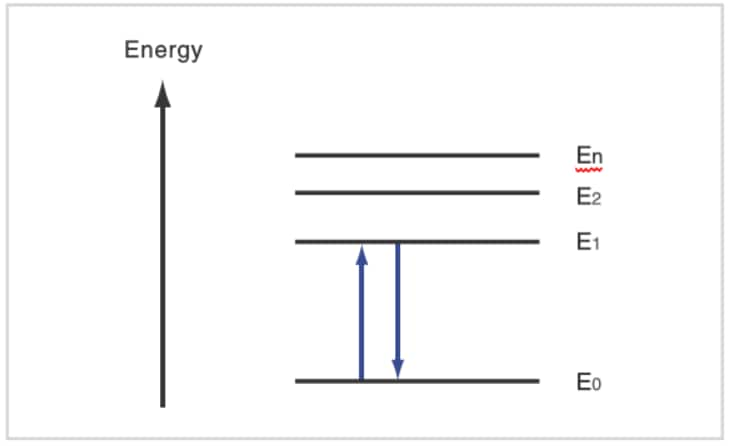# How does “absorption” spectroscopy work?Let us consider the absorption of light by matter. This is closely related to quantum mechanics. Solving the equations of quantum mechanics that relate to the electrons in an atom gives a model, like that shown here in which the electrons have discrete energy states. E0 is called the "ground state" and E1, E2, etc., are called "excited states". In order for an electron to switch from E0 to E1, light with an energy of (E1 - E0) must strike the electron. This is the "absorption" of light. Electrons have particular energy levels, and rays of ultraviolet and visible light have the energy to change the energy states of the electrons.

Because the higher energy state, E1, is unstable, the electron soon returns to the ground state, E0. The energy discharged when the electron returns from E1 to E0 (E1 - E0) is converted to heat. If, for some reason, it is not converted to heat, the energy is discharged as light. The phenomenon of light emission is well known as fluorescence or phosphorescence.

In relation to quantitative measurement performed with spectroscopy, the consequence of this phenomenon is that there is a large amount of absorption if a large number of target molecules exist in a solution, and only a small amount of absorption if there is only a small number of target molecules. Obtaining the quantity, and thereby the concentration, of a substance from the degree of absorption is the fundamental principle behind quantitative measurement.

Back to Index